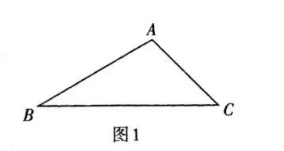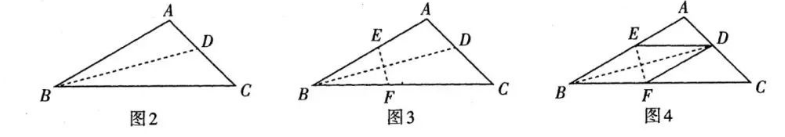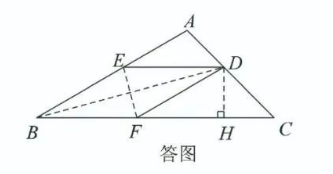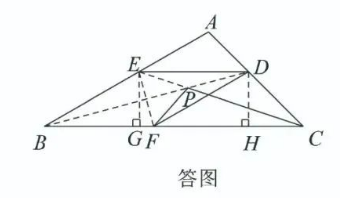A. 求四边形 $B E D F$ 的面积.
B. 设点 $P$ 在 $B D$ 上运动, 连接 $C P, P F$, 求 $C P+P F$ 的最小值.【答案】 探究一: 四边形 $B E D F$ 是菱形. 理由如下:
$\because$ 纸片沿过点 $B$ 的直线折叠, 点 $A$ 在 $B C$ 上, 折痕为 $B D$,
$\therefore \angle A B D=\angle D B C$.
$\because$ 折叠该纸片时使点 $B$ 与点 $D$ 重合,
$\therefore E F$ 是线段 $B D$ 的垂直平分线.
$\therefore E D=E B, F D=F B, E F \perp B D$.
$\therefore \angle A B D=\angle E D B, \angle D B C=\angle B D F$.
$\therefore \angle A B D=\angle B D F, \angle D B C=\angle B D E$.
$\therefore E D / / B F, B E / / D F . \therefore$ 四边形 $B E D F$ 是平行四边形.
$\because E F \perp B D, \therefore$ 四边形 $B E D F$ 是菱形.

A. 如答图, 过点 $D$ 作 $D H \perp B C$ 于点 $H$. 则 $\angle D H F=\angle D H C=90^{\circ}$.

\begin{aligned} & \therefore B F=F D, B E / / F D . \\ & \because \angle A B C=30^{\circ}, \angle A=105^{\circ}, \end{aligned}$\therefore \angle D F C=\angle A B C=30^{\circ}, \angle C=180^{\circ}-\angle A-\angle A B C=45^{\circ}$.
$\therefore$ 在 Rt $\triangle D F H$ 中, $D F=2 D H$.

$\therefore 6+2 \sqrt{3}=2 D H+\sqrt{3} D H+D H . \quad \therefore D H=2$. 7 分
$\therefore B F=D F=4$.
8 分
$\therefore$ 四边形 $B E D F$ 的面积等于 8 .
B. 如答图, 过点 $D$ 作 $D H \perp B C$ 于点 $H$, 过点 $E$ 作 $E G \perp B C$ 于点 $G$, 连接 $E C$ 交 $B D$ 于点 $P$, 连接 $P F$.
$$\therefore \angle D H F=\angle D H C=\angle E G C=\angle E G B=90^{\circ} \text {. }$$$$\therefore B F=F D=B E, B E / / F D, \quad E D / / B F \text {, }$$

$\therefore C P+P F$ 的最小值是 $C E$.
$\because \angle A B C=30^{\circ}, \angle A=105^{\circ}$,
$\therefore \angle D F C=\angle A B C=30^{\circ}, \angle A C B=180^{\circ}-\angle A-\angle A B C=45^{\circ}$

$\therefore$ 在 Rt $\triangle D F H$ 中, $D F=2 D H$.

$\therefore \angle C=\angle C D H . \therefore D H=H C$.

\begin{aligned} & \because B C=B F+F H+C H, B C=6+2 \sqrt{3} . \\ & \therefore 6+2 \sqrt{3}=2 D H+\sqrt{3} D H+D H . \therefore D H=2 . \\ & \therefore B F=D F=4 . \\ & \because E D / / B F, D H \perp B C \text { 于点 } H, \quad E G \perp B C \text { 于点 } G, \therefore E G=D H=2 . \\ & \because B E=F D=4, \angle E G B=90^{\circ}, \therefore \triangle B E G \text { 中是直角三角形. } \end{aligned}

$$\therefore C G=B C-B G=6+2 \sqrt{3}-2 \sqrt{3}=6 \text {. }$$

$$C E=\sqrt{C G^2+E G^2}=\sqrt{6^2+2^2}=2 \sqrt{10} \text {. }$$
$\therefore C P+P F$ 的最小值是 $2 \sqrt{10}$.